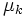# Difference between revisions of "Coulomb model of friction"

Jump to: navigation, search

## Definition

The Coulomb model of friction, or Coulomb friction, refers to a simple model of dry friction that is fairly accurate empirically. Specifically, it is a model that helps predict the direction and magnitude of the friction force between two bodies with dry surfaces in contact.

## Model for static friction

Further information: Static friction

Static friction occurs when there is no slipping between the two surfaces of contact. This can occur both when the two bodies are in rest relative to each other, and when the surfaces are rolling against each other.

There exists a constant$\mu_s$, termed the limiting coefficient of static friction, dependent on the nature of the two surfaces in contact, such that the static friction is determined by the following rules:

• Static friction is equal in magnitude and opposite in direction to the net external force acting along the plane of contact that is causing a tendency for the surfaces to slip against each other. In other words, it acts to precisely cancel out the external force that would otherwise have caused slipping, thus producing no slipping.
• The maximum value of static friction that can be experienced through the surface of contact equals$\mu_s N$, where$\mu_s$ is the limiting coefficient of static friction and$N$ is the normal force. In the limiting case, the two surfaces are said to just start slipping against each other.

The proportionality with normal force, and the fact that this constant of proportionality is dependent on the nature of the surfaces in contact, is termed Amonton's second law of friction. The fact that the magnitude of area of contact plays no role is termed Amonton's first law of friction.

## Model for kinetic friction

Further information: Kinetic friction

Kinetic friction, or dynamic friction, occurs when there is slipping between the two surfaces of contact. There exists a constant$\mu_k$, termed the coefficient of kinetic friction, dependent on the nature of the two surfaces in contact, such that, for sufficiently small speeds of slipping:

• Kinetic friction is opposite in direction to the direction of actual slipping. Its direction does not depend on the direction of other external forces.
• The magnitude of kinetic friction is$\mu_kN$, where$\mu_k$ is the coefficient of kinetic friction, and$N$ is the normal force between the bodies.$\mu_k$ for a given pair of surfaces is typically somewhat less than$\mu_s$, indicating that once two bodies start slipping against each other, it is easier for them to continue slipping.

The proportionality with normal force, and the fact that this constant of proportionality is dependent on the nature of the surfaces in contact, is termed Amonton's second law of friction. The fact that the magnitude of area of contact plays no role is termed Amonton's first law of friction. The fact that the magnitude of friction force is independent of the speed of slipping is termed Coulomb's law of friction.